## Cantor Square FractalA Fractal which can be constructed using String Rewriting by creating a matrix three times the size of the current matrix using the rules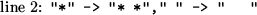The first three steps are illustrated above.

The size of the unit element after theth iteration is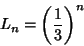and the number of elements is given by the Recurrence Relationwhere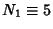, and the first few numbers of elements are 5, 65, 665, 6305, .... Expanding out gives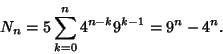The Capacity Dimension is thereforeSince the Dimension of the filled part is 2 (i.e., the Square is completely filled), Cantor's square fractal is not a true Fractal.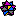Weisstein, E. W. Fractals.'' Mathematica notebook Fractal.m.